Question

# 26. For the function f(x,y) = 4y2 + 3x², find f(3. - 4), (-4,4), f(-1,-2), and...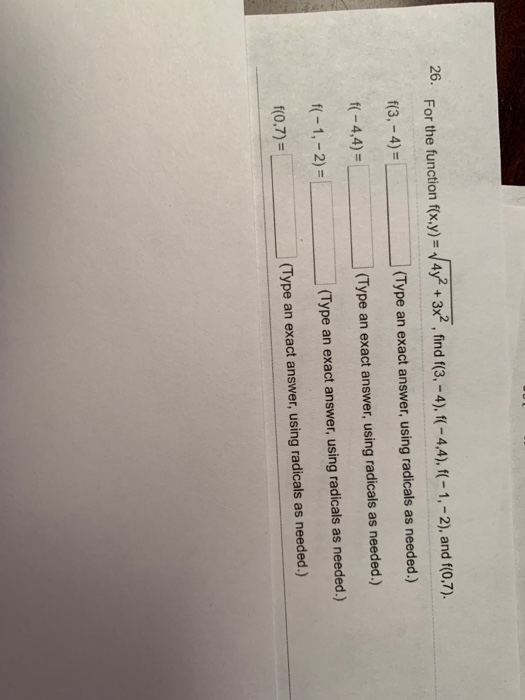26. For the function f(x,y) = 4y2 + 3x², find f(3. - 4), (-4,4), f(-1,-2), and f(0,7). f(3,-4)= (Type an exact answer, using radicals as needed.) f(-4,4)= (Type an exact answer, using radicals as needed.) f(-1, - 2) = (Type an exact answer, using radicals as needed.) f(0.7) = (Type an exact answer, using radicals as needed.)

We need at least 10 more requests to produce the answer.

0 / 10 have requested this problem solution

The more requests, the faster the answer.

All students who have requested the answer will be notified once they are available.

#### Earn Coins

Coins can be redeemed for fabulous gifts.

Similar Homework Help Questions
• ### Find the linearization L(x,y) of the function f(x,y)= e 3x cos (y) at the points (0,0)...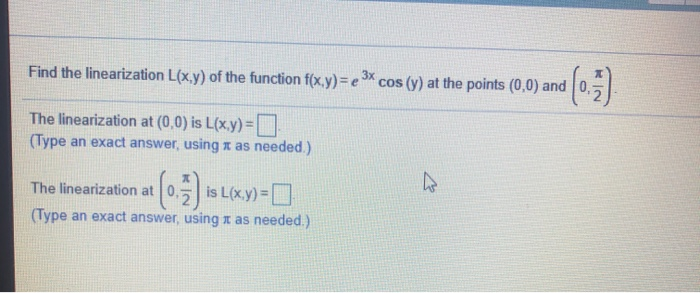Find the linearization L(x,y) of the function f(x,y)= e 3x cos (y) at the points (0,0) and RIN The linearization at (0,0) is L(x,y)= (Type an exact answer, using a as needed.) The linearization at 0. is L(x,y)= 0 (Type an exact answer, using a as needed.)

• ### Find the directions in which the function increases and decreases most rapidly at Po. Then find the derivatives of the function in these directions. xy) =x"cos(y) +x"win(x) cos(x)sin(y). Plo...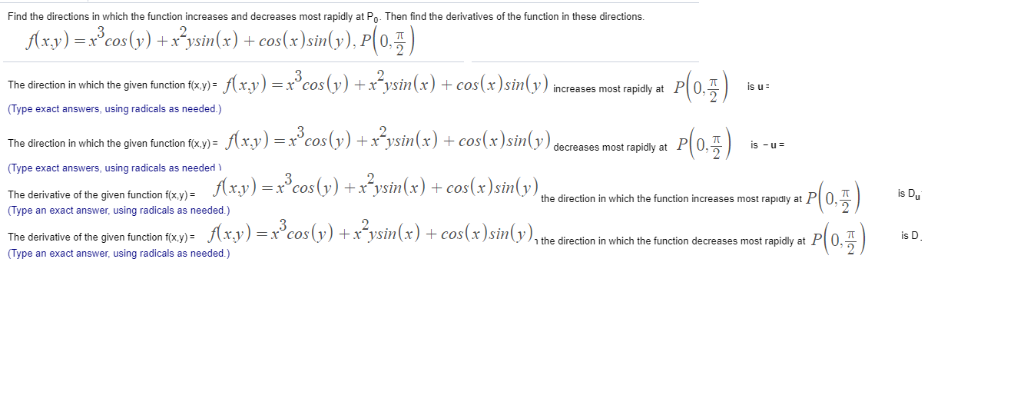Find the directions in which the function increases and decreases most rapidly at Po. Then find the derivatives of the function in these directions. xy) =x"cos(y) +x"win(x) cos(x)sin(y). Plo The direction in which the given function txy_f(xy)-x3cos(v)+x2vsin(x) + cos(x)sin(y)increases most rapidly at P 0주 is u: " (Type exact answers, using radicals as n (xy)=x3cos(y)+x"win(x)-cos(x)sin(y) The direc on in which the given function f(xy- is eases most rapidly at (Type exact answers, using radicals as needed The derivative of the...

• ### dz Consider the equation 6 sin(x + y) + 2 sin (x +z)+ sin(y +z)= 0....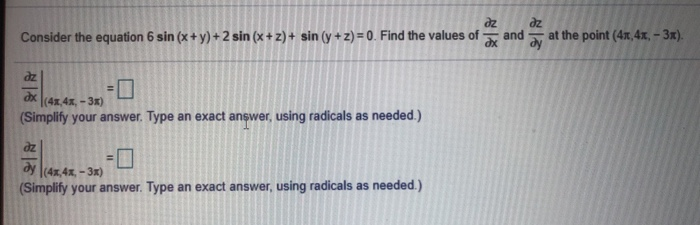dz Consider the equation 6 sin(x + y) + 2 sin (x +z)+ sin(y +z)= 0. Find the values of and dz ду at the point (41,41,- 3x). dx dz cx (Simplify your answer. Type an exact answer, using radicals as needed.) (41,4x - 3x) dz dy (43,4%, - 3x) (Simplify your answer. Type an exact answer, using radicals as needed.)

• ### Find each of the following values given that f(x)= and g(x) = 2x +1. b. (f-9)(0)...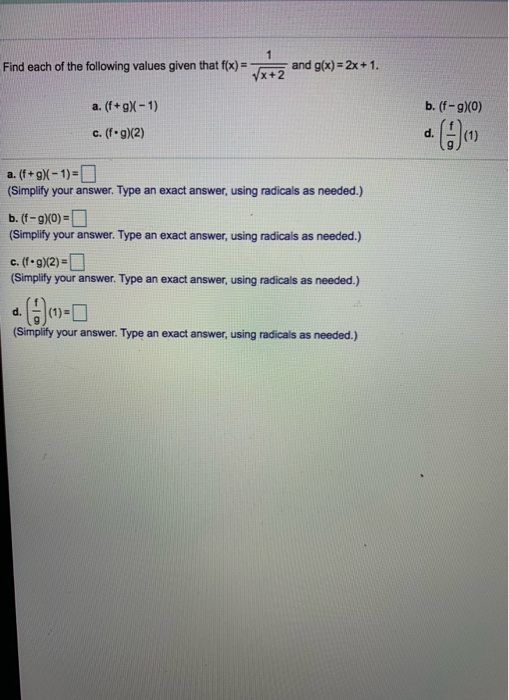Find each of the following values given that f(x)= and g(x) = 2x +1. b. (f-9)(0) a. (f+gX-1) c. (fog)(2) . (9) a. (f+9)-1)= (Simplify your answer. Type an exact answer, using radicals as needed.) b. (f-9)0)= (Simplify your answer. Type an exact answer, using radicals as needed.) C. (-9)2) = (Simplify your answer. Type an exact answer, using radicals as needed.) d. (1) (Simplify your answer. Type an exact answer, using radicals as needed.)

• ### Find the directions in which the function increases and decreases most rapidly at Po Then find...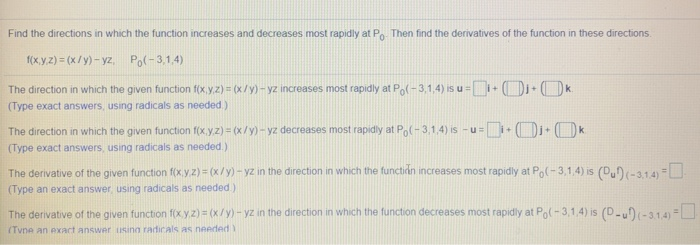Find the directions in which the function increases and decreases most rapidly at Po Then find the derivatives of the function in these directions f(x,y,z) = (x/y) - yz, Po(-3.1.4) The direction in which the given function f(x,y,z) = (x/Y)- yz increases most rapidly at Po(-3.1.4) isu = 11+( 1+ k (Type exact answers, using radicals as needed) The direction in which the given function f(x,y,z) = (x/y) - yz decreases most rapidly at Po(-3.1.4) is -u=1:-( k (Type exact...

• ### This Question: 1 pt 10 of 18 (17 complete) Find the directions in which the function...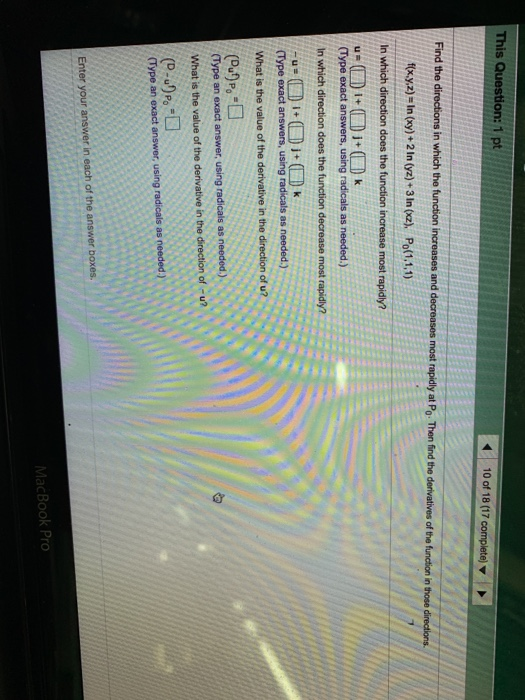This Question: 1 pt 10 of 18 (17 complete) Find the directions in which the function increases and decreases most rapidly at Po. Then find the derivatives of the function in those directions f(x,y,z) - In (xy) + 2 In (yz) + 3 In (xz). Po(1,1,1) In which direction does the function increase most rapidly? u=() i+ 1+ k (Type exact answers, using radicals as needed.) In which direction does the function decrease most rapidly? -u= (1 + ( 1+...

• ### Find the directions in which the function increases and decreases most rapidly at Po. Then find...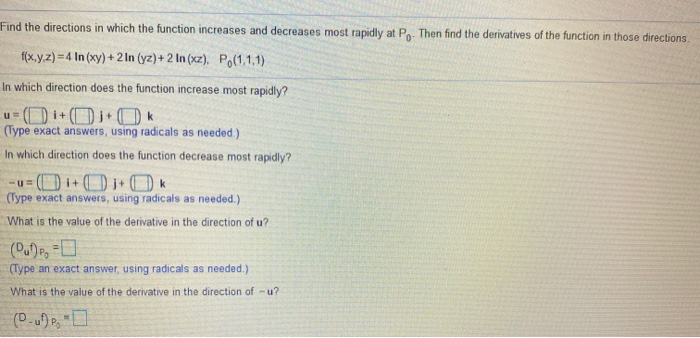Find the directions in which the function increases and decreases most rapidly at Po. Then find the derivatives of the function in those directions u f(x,y,z) = 4 In (xy) + 2 In (yz)+2 In (xz). Po(1,1,1) In which direction does the function increase most rapidly? (i+1+O (Type exact answers, using radicals as needed) In which direction does the function decrease most rapidly? = (1+) ;+ OK (Type exact answers, using radicals as needed.) What is the value of the...

• ### Find the extreme values of the function f(x, y) = 3x + 6y subject to the...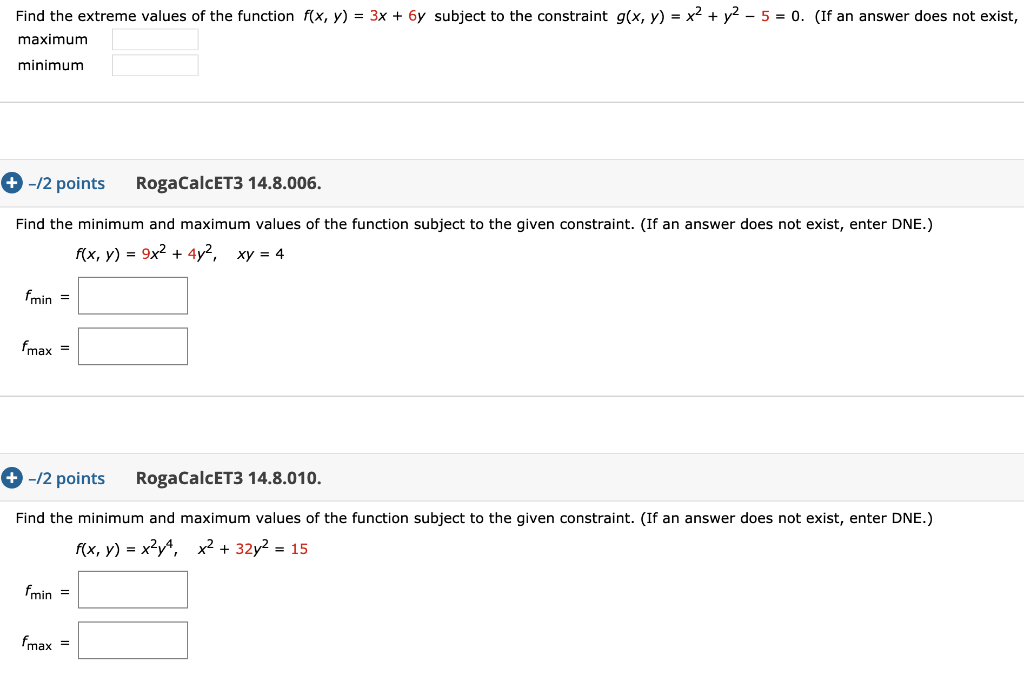Find the extreme values of the function f(x, y) = 3x + 6y subject to the constraint g(x, y) = x2 + y2 - 5 = 0. (If an answer does not exist, maximum minimum + -/2 points RogaCalcET3 14.8.006. Find the minimum and maximum values of the function subject to the given constraint. (If an answer does not exist, enter DNE.) f(x, y) = 9x2 + 4y2, xy = 4 fmin = Fmax = +-12 points RogaCalcET3 14.8.010. Find...

• ### (1 point) Consider the function f (x, y) = 3x2 + 4y2. f at the point (-4,1) in the direction given by Find the the dire...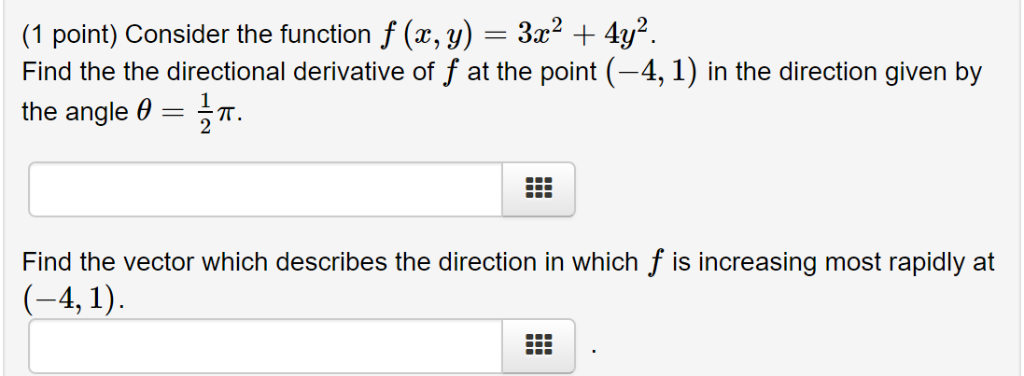(1 point) Consider the function f (x, y) = 3x2 + 4y2. f at the point (-4,1) in the direction given by Find the the directional derivative of the angle 0 Find the vector which describes the direction in which f is increasing most rapidly at (-4, 1) (1 point) Consider the function f (x, y) = 3x2 + 4y2. f at the point (-4,1) in the direction given by Find the the directional derivative of the angle 0 Find...

• ### Find Vf at the given point. f(x,y,z)=e*** cos z + (y + 2) sinx (Type an...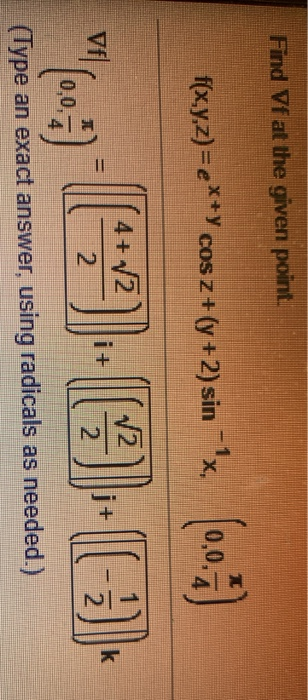Find Vf at the given point. f(x,y,z)=e*** cos z + (y + 2) sinx (Type an exact answer, using radicals as needed.)